[Next]: Interacting catalytic branching processes
 [Up]: Project descriptions
 [Previous]: Low temperature phases in models with
[Contents]   [Index]

## Gibbs measures of highly disordered systems

Collaborator: A. Bovier

Cooperation with: I. Kourkova (Université Paris VI Pierre et Marie Curie'', France)

Description: No materials in the history of solid state physics have been as intriguing and perplexing than certain alloys of ferromagnets and conductors, such as AuFe or CuMg, known as spin glasses. The attempts to model these systems have led to a class of disordered spin systems whose mathematical analysis has proven to be among the most fascinating fields of statistical mechanics over the last 25 years. Even the seemingly most simple model class, the mean-field models introduced by Sherrington and Kirkpatrick now known as SK models, haven proven to represent an amazingly rich structure that is mathematically extraordinarily hard to grasp. Theoretical physics has produced an astounding solution describing the thermodynamics properties of these models that is based on ad-hoc constructions (so-called replica symmetry breaking'' ) that so far have largely resisted attempts to be given a concrete mathematical sense. Rather than aiming at partial results in the SK model and its close relatives, we will start with the more accessible models generalizing the REM that were introduced by Derrida and Gardner , the Generalized Random Energy Models (GREM). They are characterized by the fact that the covariance of the Gaussian process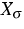on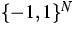is a function of the canonical ultrametric valuation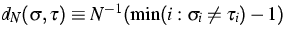rather than of the overlap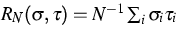, i.e.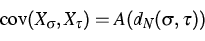where A a continuous probability distribution on [0,1] which can either be discrete (standard GREM) or have a continuous part (CREM'': GREM with continuous hierarchies'').

They form in fact a very rich class of models, in which all the phenomena expected in the SK models, such as continuous replica breaking occur. At the same time, they offer certain simplifying features that make a fully rigorous analysis feasible. In 1987, Ruelle  has suggested models based on so-called Poisson cascades that were suggested to represent the thermodynamic limits of Derrida's models. Two years ago, we have shown in the case of the simplest of these models, the REM, that the Gibbs measure converges indeed to the Gibbs measure associated to the corresponding Poisson model of Ruelle , . This year we have extended this result to the full class of Derrida's models, including those with continuous hierarchies (, , ). This provides for the first time examples of models in which all of the rather mysterious'' features of Parisi's solution of mean-field spin glasses arise and can be understood in a precise mathematical way.

A major motivation for this work is that these results provide the basis for the analysis of the dynamics of these models. Such an analysis has already been carried out in the case of the REM , but we are now hopeful that similar results can be obtained for the far more complex models studied here.

References:

1. A. BOVIER, Statistical mechanics of disordered systems, MaPhySto Lecture Notes, 10 (2001), Aarhus.
2. A. BOVIER, G. BEN AROUS, V. GAYRARD, Aging in the random energy model, Phys. Rev. Lett., 88 (2002), pp. 087201/1-087201/4.
3. A. BOVIER, I. KOURKOVA, Derrida's generalized random energy models. 1. Poisson cascades and extremal processes, Preprint no. PMA-727, Université Paris VI Pierre et Marie Curie'', Paris, 2002, submitted.
4.,Derrida's generalized random energy models. 2. Gibbs measures and probability cascades, Preprint no. PMA-728, Université Paris VI Pierre et Marie Curie'', Paris, 2002, submitted.
5.,Derrida's generalized random energy models. 3. Models with continuous hierarchies, Preprint no. PMA-729, Université Paris VI Pierre et Marie Curie'', Paris, 2002, submitted.
6. A. BOVIER, I. KOURKOVA, M. LÖWE, Fluctuations of the free energy in the REM and the p-spin SK-models, Ann. Probab., 30 (2002), pp. 605-651.
7. B. DERRIDA, E. GARDNER, Solution of the generalized random energy model, J. Phys. C, 19 (1986), pp. 2253-2274.
8. M. MÉZARD, G. PARISI, M.A. VIRASORO, Spin-glass Theory and Beyond, World Scientific, Singapore, 1988.
9. D. RUELLE, A mathematical reformulation of Derrida's REM and GREM, Comm. Math. Phys., 108 (1987), pp. 225-239.

 [Next]: Interacting catalytic branching processes
 [Up]: Project descriptions
 [Previous]: Low temperature phases in models with
[Contents]   [Index]

LaTeX typesetting by I. Bremer
5/16/2003